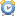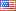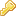Product:Genetic calculator Version:4.0 final File size:14.3 Mb Language:En / Ru License:Free OS:Win XP/Vista/7/8

## Punnett square practice and examples

### Punnett square definition

As is well known make a punnett square is widely used for solving genetics problems in mendelian genetics. An ability to make a punnett squares will be useful for middle and high school students in biology classes. But professional geneticists use these skills in their work too. So what is punnett square?

Punnett Square - is a graphical method proposed by the British geneticist R. Punnett in 1906 to visualize all the possible combinations of different types of gametes in particular crosses or breeding experiments (each gamete is combination of one maternal allele with one paternal allele for each gene being studied in the cross).

Punnett Square looks like a two-dimensional table, where over the square horizontally fit the gametes of one parent, and the left edge of the square in the vertical - the gametes of the other parent. Within the square, at the intersection of rows and columns, write genotypes making from the gametes combinations. Thus, it becomes very easy to determine the probability of each genotype in a particular cross.

### Monohybrid punnett square

For monohybrid cross we study the inheritance of a single gene. In the classical monohybrid cross each gene has two alleles. For example, to make our punnett square, we take the maternal and paternal organisms with the same genotype - "Gg". For dominant allele in genetics we use upper-case letters and for recessive allele lower-case letters. This genotype can produce only two types of gametes that contain either the "G" or "g" allele.

So this Punnett square look like that:

 G g G GG Gg g Gg gg

From punnett square in the offspring we have genotype ratio and probability: 1(25%)GG : 2(50%)Gg : 1(25%)gg - this typical genotypes ratio (1:2:1) for a monohybrid cross.Dominant allele will mask the recessive allele that means, that the organisms with the genotypes "GG" and "Gg" have the same phenotype.

For example, if allele "G" is yellow color and allele "g" is green color, then "gg" have green phenotype, "GG" and "Gg" have yellow phenotype. From punnett square in the amount we have 3G-(yellow phenotype) and 1gg(green phenotype) - this typical phenotypes ratio (3:1) for a monohybrid cross. And probability of the offspring will be 75%G- : 25%gg.

### Mendelian genetics

At first these results were obtained in the experiments of Gregor Mendel to the plant - garden pea (Pisum sativum). To expound the results Gregor Mendel made the following conclusions:

• Every trate of this organism is controlled by a pair of alleles.
• If the organism contains two different alleles for this trait, then one of them (the dominant) can manifest itself, completely suppressed the manifestation of the other (recessive).
• During meiosis, each pair of alleles split(segregate), and each gamete receives one allele from each pair of alleles the principle of segregation.

Without this basic genetics lows we can't solve any punnett square problems. Determine possibility to predict the results of one pair of alternative traits, Mendel went on to study the inheritance of two pairs of such traits.

### Dihybrid punnett square

For dihybrid cross we study the inheritance of two genes. For dihybrid cross the Punnett squares only works if the genes are independent of each other, which meomans when form a maternal and paternal gametes - each of them can get any allele of one pair, along with any allele of the another pair. This principle of independent assortment was discovered by Mendel in experiments on dihybrid and polyhybrid crosses.

The following example illustrates Punnett square for a dihybrid cross between two heterozygous pea plants. We have two genes Shape and Color. For shape: "R" is dominant allele with round phenotype and "w" is recessive allele with wrinkled phenotype. For color: "Y" is dominant allele with yelloy phenotype and "g" is recessive allele whith green phenotype. Maternal and paternal organisms have some genotype- "RwYg".

First you need to determine all possible combinations of gametes, for this you can also use Punnett squares:

 R w R RR Rw w Rw ww

Then they can produce four types of gametes with all possible combinations: RY, Rg, wY, wg. And now form the Punnett square for genotypes:

 RY Rg wY wg RY RRYY RRYg RwYY RwYg Rg RRYg RRgg RwYg Rwgg wY RwYY RwYg wwYY wwYg wg RwYg Rwgg wwYg wwgg

From punnett square in the offspring we have genotype ratio and probability: 1(6,25%)RRYY : 2(12,5%)RwYY : 1(6,25%)wwYY : 2(12,5%)RRYg : 4(25%)RwYg : 2(12,5%)wwYg : 1(6,25%)RRgg : 2(12,5%)Rwgg : 1(6,25%)wwgg.

Since dominant traits mask recessive traits, from punnett square we have phenotypes combinations whith ratio and probability: 9(56,25%)R-Y-(round, yellow) : 3(18,75%)R-gg(round,green) : 3(18,75%)wwY-(wrinkled, yellow) : 1(6,25%)wwgg(wrinkled, green). The ratio 9:3:3:1 is typical for a dihybrid cross.

### Trihybrid punnett square

Make punnett square for trihybrid cross between two heterozygous plants is more complicated. To solve this problem, we can use our knowledge of mathematics. To determine all possible combinations of gametes for trihybrid cross we have to remember the solution of polynomials:

• Let make polynomial for this cross: (A + a) X (B + b) X (C + c).
• We multiply the expression in the first bracket on the expression of a second and we get : (AB + Ab + aB + ab) X (C + c).
• Now multiply this expression by the expression in the third bracket and we get: ABC + ABc + AbC + Abc + aBC + aBc + abC + abc.

Then they can produce eight types of gametes with all possible combinations. This solution can be illustrated by the Punnett square:

 A a B AB aB b Ab ab

 C c AB ABC ABc Ab AbC Abc aB aBC aBc ab abC abc

Now form the Punnett square for genotypes (we get 64 punnett square):

 ABC aBC AbC abC ABc aBc Abc abc ABC AABBCC AaBBCC AABbCC AaBbCC AABBCc AaBBCc AABbCc AaBbCc aBC AaBBCC aaBBCC AaBbCC aaBbCC AaBBCc aaBBCc AaBbCc aaBbCc AbC AABbCC AaBbCC AAbbCC AabbCC AABbCc AaBbCc AAbbCc AabbCc abC AaBbCC aaBbCC AabbCC aabbCC AaBbCc aaBbCc AabbCc aabbCc ABc AABBCc AaBBCc AABbCc AaBbCc AABBcc AaBBcc AABbcc AaBbcc aBc AaBBCc aaBBCc AaBbCc aaBbCc AaBBcc aaBBcc AaBbcc aaBbcc Abc AABbCc AaBbCc AAbbCc AabbCc AABbcc AaBbcc AAbbcc Aabbcc abc AaBbCc aaBbCc AabbCc aabbCc AaBbcc aaBbcc Aabbcc aabbcc

#### Genotypes ratio and probability for Trihybrid cross

But how do we calculate the ratio of genotypes from this punnett square. Again use the polynomials.

• We know the genotype ratio for monohybrid cross: 1AA: 2Aa: 1aa.
• Now we form a polynomial for our case: (1AA + 2Aa + 1aa) X (1BB + 2Bb + 1bb) X (1CC + 2Cc + 1cc).
• Multiply the first two expressions we get : (1AABB + 2AABb + 1AAbb + 2AaBB + 4AaBb + 2Aabb + 1aaBB + 2aaBb + 1aabb) X (1CC + 2Cc + 1cc).
• Multiplying this expression on the third we get the results for genotypes : 1AABBCC : 2AABbCC : 1AAbbCC : 2AaBBCC : 4AaBbCC : 2AabbCC : 1aaBBCC : 2aaBbCC : 1aabbCC : 2AABBCc : 4AABbCc : 2AAbbCc : 4AaBBCc : 8AaBbCc : 4AabbCc : 2aaBBCc : 4aaBbCc : 2aabbCc : 1AABBcc : 2AABbcc : 1AAbbcc : 2AaBBcc : 4AaBbcc : 2Aabbcc : 1aaBBcc : 2aaBbcc : 1aabbcc

#### Phenotypes ratio and probability for Trihybrid cross

And as well we can calculate from our punnett square the ratio of phenotypes.

• We know the phenotype ratio for monohybrid cross: 3A-: 1aa.
• Now we form a polynomial for our case: (3A- + 1aa) X (3B- + 1bb) X (3C- + 1cc).
• Multiply the first two expressions we get : (9A-B-+ 3A-bb + 3aaB-+ 1aabb) X (3C-+ 1cc).
• Multiplying this expression on the third we get the results for phenotypes : 27A-B-C- : 9A-bbC- : 9aaB-C- :3aabbC- : 9A-B-cc : 3A-bbcc : 3aaB-cc : 1aabbcc

All this results we can get from polinomial method, without making punnett squares.

### How to solve a large and complicated punnett square examples

But what should we do if we need to solve the problem with a large number of genes. Even using the polynomials will be difficult to avoid mistakes and get the right results. In addition it may be time-consuming. And if you do not want to do all this job manually, then you can use our professional Punnett Square Calculator.